# What is Analytical Rheology?

Analytic rheology is the subject of determining the material’s microstructure based on the measurements of its viscoelastic response.

It is an extension of analytic chemistry in a way similar to other analytic methods predicated on flow properties. One example of the analytical technique that comes under this category is intrinsic viscosity.

Analytic rheology can be used on any material system while the rheological response is based on the microstructure. There are different examples of such rheology-based systems. For instance, based on the measured linear viscoelastic response, the droplet size distribution of a multiphase suspension can be determined. Experimental methods are highly evolved to determine the linear viscoelastic material functions so as to make accurate and reliable measurements in an automated manner. Analytic rheology makes use of this experimental capability to develop advanced interpretation methods. In advanced data interpretation methods such as MWD determinations, the TAOrchestrator software allows the full power of rheological characterization methods. The calculation of the molecular weight distribution for linear flexible polymers based on the measured linear viscoelastic material functions is the specific application of analytic rheology discussed in this article.

## Benefits of Rheological MWD Determination

It is essential to identify the advantages of having a rheological MWD determination method as there are a number of viable methods available for determining the molecular weight distribution of flexible polymers, including intrinsic viscosity, light scattering, gel permeation chromatography, etc.

There are several advantages to rheologically-based methods for determining the molecular weight distribution. For instance, virtually all conventional MWD methods are predicted based on the ability to readily dissolve the polymer in a solvent at room temperatures. However, most of the commercially used polymers including polypropylene, polyethylene and Teflon are slightly soluble in common solvents at room temperatures. This prevents access to standard molecular weight determination methods used for commercially important polymer systems.

Rheological methods avoid the need for such time consuming solvating procedures. In addition, there are no specific experimental difficulties in achieving rheological data for polyethylene or polypropylene melts. The fact that the rheological methods are necessarily secondary analytic methods which demand a primary analytic method for calibration should also be considered. Upon using MWD methods for these polymers, the resolution and sensitivity of the analytic techniques is poor, particularly for the high molecular weight tail.

Characterization of the high molecular weight tail is critical for the characterization of the processability of a commercially available polymer. Rheological methods used for MWD determination are intrinsically sensitive to the high end of the molecular weight distribution, which is evident from the dependence of the linear viscoelastic material functions on molecular weight and molecular weight distribution. For instance, the zero shear viscosity depends on the weight average molecular weight to the 3.4 power. Therefore, any small changes in weight average molecular weight can lead to large changes in zero shear viscosity.

Only a small portion of the linear viscoelastic spectrum consists of information on the molecular weight of the system. These concepts are based on the Bueche-Ferry /1/ theorem. According to the Bueche-Ferry hypothesis, the response of all flexible polymers is similar on sufficiently short time scales, irrespective of the polymer concentration, chain architecture or molecular weight. This shows that the viscoelastic response in the glassy regime is not affected by the MWD. This result is obtained from the fact that the entanglement effect can be observed on relatively large length scales when compared to typical monomeric dimensions.

Eventually, the mechanical response’ dependence on molecular weight is completely lost in the glassy time/ frequency range. Different regimes of dynamic response for a monodisperse polymer melt are shown in Figure 1. The availability of the “glassy modes” in data sets demands a suitable way of choosing these effects out of the experimental data before MWD calculation. The principal complication, however, is that the lower Rouse modes for the high molecular weight components can be combined with repetitive relaxation modes for the lower molecular weight species.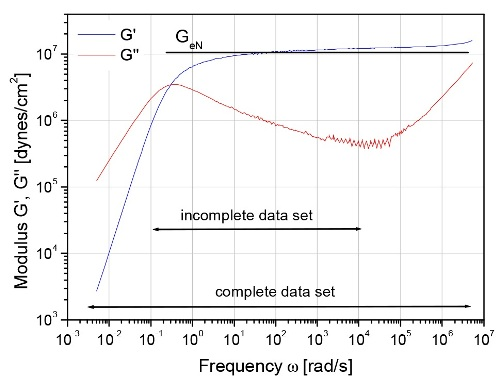Figure 1. Dynamic responses of a monodisperse linear flexible polymer are identical to those of a monomer in a larger or shorter chain, or a polymer in dilute solution for that matter

## Rules for Mixing Polymer Melts

A mixing rule is a quantitative relationship between the observed mechanical properties of a polydisperse melt and the underlying microstructure. Tsenoglou and des Cloizeaux recently derived a viable mixing rule for homogeneous systems of well entangled polymers. The mixing rule known as the “double reptation” model is a relatively simple mathematical approximation to a more rigorous and complex molecular theory of polydispersity. The mathematical structure for the double reptation mixing rule is as follows: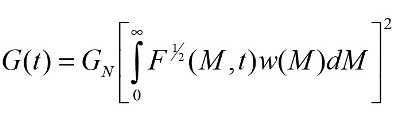(1)

where G(t) is the relaxation modulus that can be determined using different combinations of linear viscoelasticity experiments given below. The F1/2 (M,t) is the monodisperse relaxation function representing the time dependent fractional stress relaxation of a monodisperse polymer following a small step strain. w(M) is the weight-based molecular weight distribution. It can be said that the components of the molecular weight distribution will add to the modulus to an extent. This concept is applied to the integral over the molecular weight distribution w(M) which sums contributions from each component of the MWD to G(t) weighted by the kernel function F1/2 (M,t). This describes the “mixing” effect where one component of a complex molecular weight distribution dynamically interacts with all of its neighbors. The TAOrchestrator software consists of options to function under the so called “weight average” mixing rule derived by Marin et al.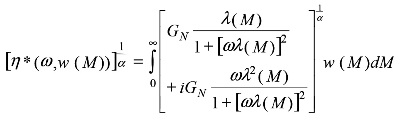(2)

The weight average mixing rule (2) is originally empirical. The physical basis for this mixing rule is determined based on the empirical observation that the relaxation time scales as molecular weight to ~3.4 power coupled with dimensional analysis.

## Material Dependent Input Parameters

The molecular weight distribution for a given material can be calculated using equation (1) for which the material dependent data need to be provided for the application. Particularly, it is necessary to provide the plateau modulus GN and the form of the monodisperse relaxation function F1/2(M,t). The plateau modulus is however tabulated in several references. The monodisperse relaxation function can be used in several forms. In general, the following single exponential form can be used.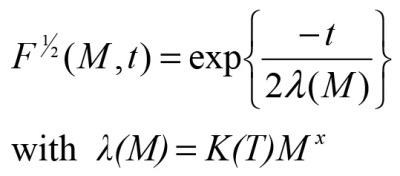(3)

where λ(M) is the characteristic relaxation time for the monodisperse system and K(T) is a temperature-dependant coefficient. For flexible polymers, the exponent x is typically ~3.4. Furthermore, factors like monodisperse relaxation function such as the Doi-Edwards could also be used.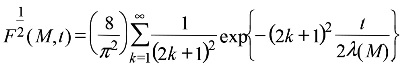(4)

When the accuracy of the experimental data does not meet the expectation, the significant differences in the predicted MWD from equation 1 can be discerned using equation (3) or (4). Based on an Arhenius type activation energy, the temperature dependence of K(T) is modeled neglecting the availability of other options like WLF. Data for the material dependent parameters can be obtained from standard references like Ferry or the research literature. Experiment and calibration may be required for novel polymers.

## Calculation of Relaxation Modulus Based on Linear Viscoelasticity Data

The relaxation modulus G(t) can be determined from linear viscoelasticity data using several methods. All linear viscoelastic material functions may include equivalent information. However, some of the linear viscoelastic material functions are intrinsically sensitive to short and long time-scales when compared to others. Constrained elastic recovery experiments, for example, are sensitive to long time-scale relaxation processes whereas the dynamic moduli measurements are sensitive to short time-scale relaxation processes. The TAOrchestrator software provides a suitable way of combining these data to enable expansion of the effective dynamic range of the relaxation modulus determination. In general, incorporation of linear viscoelasticity data from different experiments is beneficial to achieve a self-consistent determination of the relaxation modulus over a wide dynamic range.

As discussed earlier, the linear viscoelasticity data on the plateau and terminal regions may contain information related to the molecular weight and molecular weight distribution. Hence, after calculating a relaxation modulus, the short time-scale contributions due to the glassy modes can be eliminated. The TAOrchestrator software achieves this by estimating the MWD. Using the estimated MWD, the Rouse-like glassy response can be calculated by adding the all Rouse modes for all components of the MWD and by subtracting it from G(t). In order to calculate a molecular weight distribution by experimentally determining the relaxation modulus, there are possibilities of achieving two distinct experimental situations such as complete dynamic moduli data and incomplete dynamic moduli data. A data set is said to be complete when the data spans a dynamic time/frequency range from fully terminal behavior through transition to the glassy modes as shown in Figure 1. Research applications may require complete data sets. However, considering the practical circumstance of characterizing of commercial polymers with broad molecular weight distribution, incomplete” data sets are preferred. In such cases, it is impossible to achieve either fully terminal behavior due to exceptionally long relaxation times of the high molecular weight tail. Each of the cases described above have different computational issues which result in restrictions to the method that we explore below.

## Complete Dynamic Moduli Data Sets

It is necessary to make use of all rheological information for inverting the mixing rule in the presence of dynamic moduli data from the terminal region through the plateau region. The problem at this stage is to invert the double reptation model for the MWD in a stable and robust manner. The double reptation mixing rule is a Fredholm integral equation of the first kind for W(M). There are well-established methods for solving these problems. Based on the regularization methods used for the moments of the MWD calculated from the experimental data through Mellin transforms, the molecular weight distribution can be calculated. The result is a sturdy and stable numerical method.

## Incomplete Dynamic Moduli Data Sets

The data set is incomplete, and the double reputation model cannot be inverted without additional information unless the experimental data span the entire frequency range from the terminal to the plateau region.

Prior information on the shape of the distribution can be added into the numerical algorithm. The method involved is assumed to have prior information about the shape of the molecular weight distribution. This assumption is valid for all commercial polymers where the polymer catalyst chemistry determines the MWD shape.

The numerical method effectively fits the predicted dynamic moduli curve from model molecular weight distributions to the measured experimental data in an optimal manner. The method involves manipulating a candidate MWD to obtain an optimal fit to the rheological data. In addition, a number of model molecular weight distributions can be tried along with binary combinations.

The accuracy of the fit to the measured data can be improved by increasing the number of fitting parameters. However, most of the commercial polymers model molecular weight distributions or combinations were observed to have satisfactory performance. The TAOrchestrator software facilitates automated fitting or a user defined manual fit to the data. This feature enables the user to achieve precise fits to the particular region of the data desired by overriding the software.

The TAOrchestrator software consists of two types of model molecular weight distribution. The first is a Wesslau or log normal molecular weight distribution that indicates the addition polymers or polymers produced using Zeigler-Natta catalyst systems. The second is a Schultz distribution, a generalized most probable molecular weight distribution consisting of more number of polymers produced through condensation reactions or using metallocene catalysts.

## Applications of Dynamic Moduli Sets

The dynamic moduli and calculated MWD for a bidisperse system of polybutadiene are shown in Figures 2 and 3. The dynamic moduli data set is complete, and the MWD calculated using the complete data set method was observed to have overlapped with the MWD measured using GPC. However, the rheological MWD and the GPC data were found to be in good agreement with each other.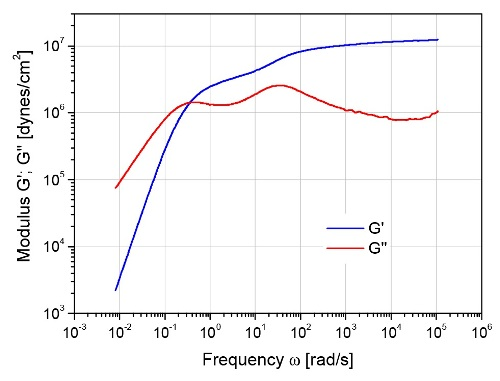Figure 2. Dynamic response of a bidisperse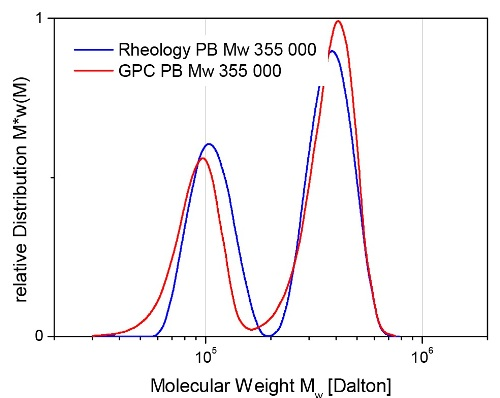Figure 3. Calculated MWD of a bidisperse polybutadiene blend

The two metallocene catalyzed binary polyethylene blends are shown in Figure 4. The dynamic moduli data sets are incomplete either in the plateau region or terminal region. Figure 5 shows the results of using the incomplete data set method with a combination of two Schultz model molecular weight distributions. The agreement is also good which indicates the viability of MWD methods for applications in commercial systems.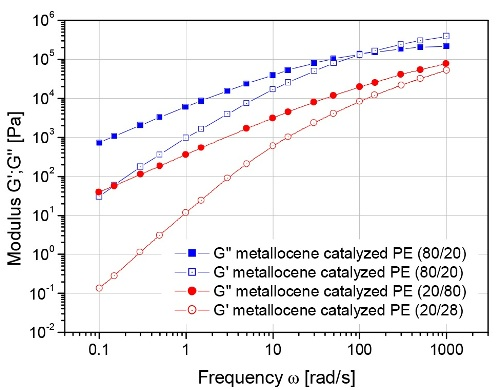Figure 4. Dynamic response of a binary polyethylene blend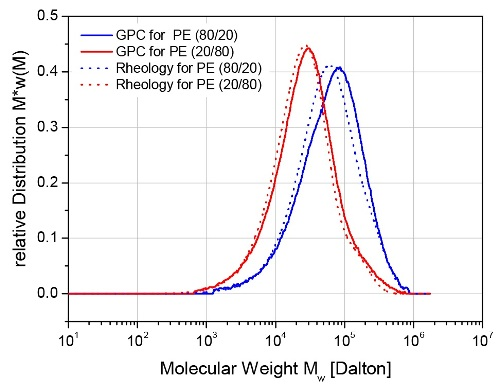Figure 5. Calculated MWD of a binary polyethylene blendThis information has been sourced, reviewed and adapted from materials provided by TA Instruments.

## Citations

• APA

TA Instruments. (2020, April 28). What is Analytical Rheology?. AZoM. Retrieved on June 05, 2020 from https://www.azom.com/article.aspx?ArticleID=12102.

• MLA

TA Instruments. "What is Analytical Rheology?". AZoM. 05 June 2020. <https://www.azom.com/article.aspx?ArticleID=12102>.

• Chicago

TA Instruments. "What is Analytical Rheology?". AZoM. https://www.azom.com/article.aspx?ArticleID=12102. (accessed June 05, 2020).

• Harvard

TA Instruments. 2020. What is Analytical Rheology?. AZoM, viewed 05 June 2020, https://www.azom.com/article.aspx?ArticleID=12102.